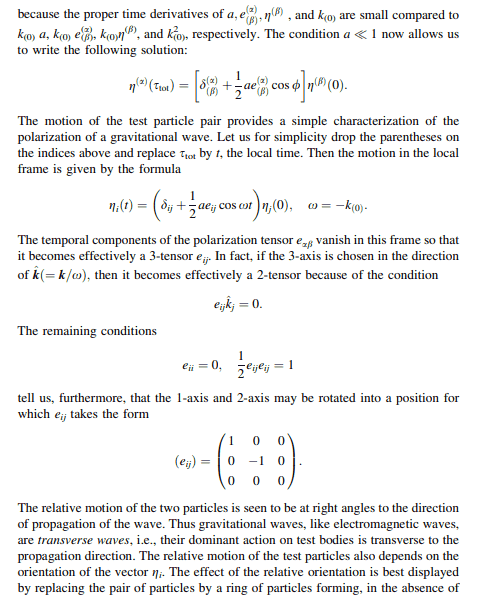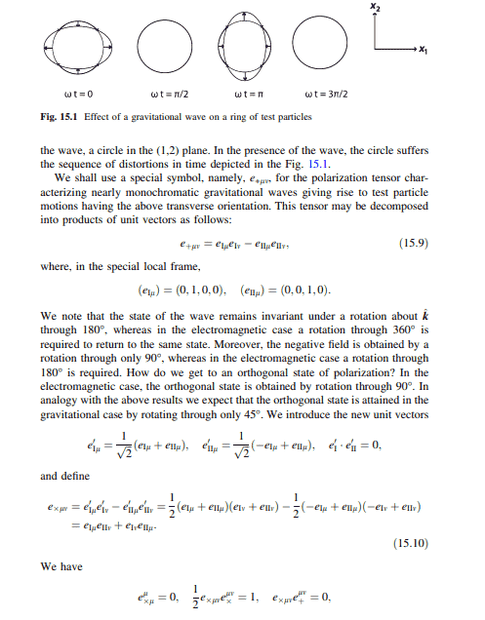# Quadrupole formula - polarization tensor

• A
Staff Emeritus
In "Gravitational radiation from point masses", by Peters & Mathews, http://gravity.psu.edu/numrel/jclub/jc/Peters_Mathews_PR_131_435_1963.pdf, the emitted power from gravitatioanal quadrupole radiation per unit solid angle ##\Omega## is given by:

$$\frac{dP}{d\Omega} = = \frac{ G} {8 \pi c^2 } \left( \frac { d^3 Q_{ij} } { dt^3} {e}^{ij} \right)$$

I'm somewhat familiar with the quadrupole moment Q_{ij}, but not the polarization tensor ##e_{ij}##. Any pointers as to how this tensor is defined and/or where I can find more information? I don't recall reading about this tensor in any of my texts, but it seems to be important in reducing the tensor quantity resulting from the 3d time derivative of Q_{ij} into a scalar that can be squared, then summed to give the total emitted power in gravitational radiation from the power/unit angle.

romsofia
Very old paper, and you rarely see it applied in relativity, but of course you can.

Things like ##e_{ij}## are known as polarization tensor of the wave (as you said). It comes from the Eikonal approximation, which you rarely see used in GR, so I only know of one person who uses it, the underrated relativist Bryce DeWitt! You can find this approximation used in GREAT detail in his lectures notes on gravitation.

EDIT: Specifically, chapter 15 on gravitational waves. And also, if you can't get access to the book, let me know and I can put more details in this thread for you. If you want more math, I can into that. If you want the physical intuition, I can try my best to explain that as well.

Staff Emeritus
The first goal is to make sure I have the components of the polarization tensor correct. Let us choose spherical coordinates (r, ##\theta##, ##\phi##) and radiation in the ##\hat{r}## direction, the "hat" over the r denoting a unit vector (same notation as the author, I believe). Then what I'd expect is that we replace the unit vectors ##\hat{x}## and ##\hat{y}## for radiation in the ##\hat{z}## direction with the unit vectors ##\hat{\theta}## and ##\hat{\phi}##. Performing a direct substitution in the expressions for e1 and e2, I get:

$$e1 = \frac{1}{\sqrt{2}} \left( \hat{\phi}\hat{\phi} - \hat{\theta}\hat{\theta} \right) \quad e2 = \frac{1}{\sqrt{2}} \left( \hat{\phi} \hat{\theta + \hat{\theta} \hat{\phi}} \right)$$

Furthermore I'm assuming that ##Q_{ij}## as well as the polarization tensors are 3-tensors based on the remarks about "working in a gauge where ##e_{\mu\nu}## is spacelike.

Does this look OK so far? I'm also rather puzzled about how the author gets from 3&4 to 5, but this may just be a matter of grinding things through from the above assumptions.

Finally, any insight would be appreciated. I don't quite see why we should have to assume a specific basis to get a tensor expression for the radiated power in a unit angle. Physically, choosing ##\hat{r}## and ##\hat{t}## makes sense, I have no problem with that. The bivector orthogonal to (r,t) is well defined as well. But in the calculation for power, we wind up assuming a specific choice of basis vectors for the spatial plane orthognal to (r,t). Using these specific basis vectors, we finding the power in the two polarizations, which are basis dependet because the polarizations are basis dependent, then sum both powers to get the total power, which should be basis independent. The process seems a bit awkwards, and not in the spirit of coordinate independence, though perhaps it is simply convenient. It's not clear to me what the alternative might be, though.

•romsofia
romsofia
Those look correct, my copy of his notes are in my office, and I'll respond in more detail tomorrow.

romsofia
Finally, any insight would be appreciated. I don't quite see why we should have to assume a specific basis to get a tensor expression for the radiated power in a unit angle. Physically, choosing ##\hat{r}## and ##\hat{t}## makes sense, I have no problem with that. The bivector orthogonal to (r,t) is well defined as well. But in the calculation for power, we wind up assuming a specific choice of basis vectors for the spatial plane orthognal to (r,t). Using these specific basis vectors, we finding the power in the two polarizations, which are basis dependet because the polarizations are basis dependent, then sum both powers to get the total power, which should be basis independent. The process seems a bit awkwards, and not in the spirit of coordinate independence, though perhaps it is simply convenient. It's not clear to me what the alternative might be, though.
So, I don't have as much time as I would like to review the topic as I would've liked (and I've forgotten more than I'd like to admit on the topic), and since it's 4th of July this weekend, even less likely I'll have time to write it up until next week, but I do think I will write something about this in my lecture notes! So I do appreciate this thread for that reason. But, I'll have to think about what the alternative would be as well. Bryce DeWitt's notation is always an issue to work through, but he does go through the math in both the E+M wave case, and gravitational wave case. However, to get to the section on this in his book, you have to slog through all his math.

However, that won't be of use to you until they're done. For the meantime (or until you can access his notes), hopefully this can hold you over:•pervect